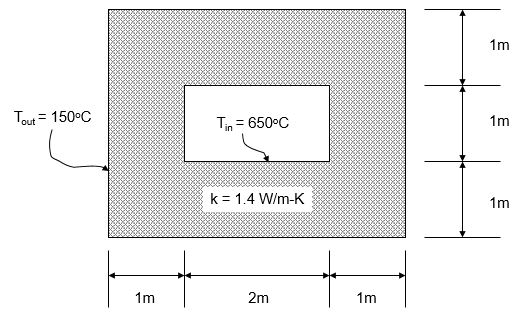### Create an Account

Home / Questions / A symmetrical furnace wall has the dimensions shown with isothermal inner and outer wall t...

# A symmetrical furnace wall has the dimensions shown with isothermal inner and outer wall temperatures as given below Find 1 the temperature profile through the wall use one quadrant and a

A symmetrical furnace wall has the dimensions shown with isothermal inner and outer wall temperatures as given below.

Find: (1) the temperature profile through the wall (use one quadrant and a minimum of 6 node points across a wall, thus the total number of nodes > 90). The calculated temperatures must be obtained by computer and should be reported in tabular form as well as graphical. To increase the grid points, you may choose to solve just one quadrant. Note that the end walls of the quadrant will be adiabats. A computer solution must be obtained.

(2) the heat flow through the entire wall surface (e.g., evaluate the heat transfer at either the inner or outer surface at each surface node and then obtain the sum over all the surface nodes).

(3) provide examples of the nodal equations used in your numerical solution.Show transcribed image text A symmetrical furnace wall has the dimensions shown with isothermal inner and outer wall temperatures as given below. Find: (1) the temperature profile through the wall (use one quadrant and a minimum of 6 node points across a wall, thus the total number of nodes > 90). The calculated temperatures must be obtained by computer and should be reported in tabular form as well as graphical. To increase the grid points, you may choose to solve just one quadrant. Note that the end walls of the quadrant will be adiabats. A computer solution must be obtained. (2) the heat flow through the entire wall surface (e.g., evaluate the heat transfer at either the inner or outer surface at each surface node and then obtain the sum over all the surface nodes). (3) provide examples of the nodal equations used in your numerical solution.

Jun 19 2020 View more View LessSubscribe To Get Solution